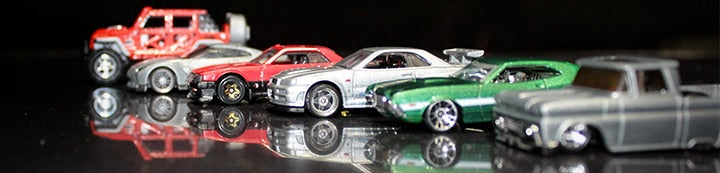1 - 2 of 2 Posts

#### oggy4u

·
##### Registered
Joined
·
67 Posts
Discussion Starter
Hi,
I am trying to put 3 LED's ,same type white , along with an SMD into the warp Nacelle of a Bandai Voyager model . The available space inside the nacelle is quite tight . I am using a 360ohm resistor on a 9 volt source . I hooked up all 4 LED's and it seems to work fine but as a beginner in electronics I want to know if I should put a resistor on each LED ? Any advice ,please .

#### Alien

·
##### Registered
Joined
·
557 Posts
That is a bit of a loaded question as it depends on a number of things.
You can certainly use one resistor to run multiple LEDs but the LEDs should be the same type, otherwise some may get too much voltage supplied to them.
The resistor must also be of a high enough wattage rating to cope with current.

Let's take an example.
Four LEDs connected in parallel via one resistor to 9 volts.
Let's assume that the forward voltage of the LEDs is 3 volts and the forward current is 20 mA.
The four LEDs in parallel would consume 4 x 20mA = 80mA.

The calculation for the dropping resistor is (9-3)/.08 = 75 ohms
This resistor makes the LEDs light up to their max brightness. You are using a 360 ohm resistor so your LEDs will be dimmer.

The 75 ohm resistor will have 6 volts across it and 80 mA passing through it.
Volts X Amps = Watts so 6 x .08 = .48watts. So you would need at least a half watt 75 ohm resistor.

So in your situation with a 360 ohm resistor, the current will be reduced significantly so you could get away with a smaller wattage resistor but I would still use a half watt resistor to be safe.

If you have a multimeter then you can check your circuit. Measure the current consumption of your 4 LEDs with resistor on your 9 volt power source. Measure the voltage across your 360 ohm resistor. Multiply the two numbers together to work out the wattage and make sure your resistor is rated above that wattage.

Hope that this is clear.

Alien

1 - 2 of 2 Posts